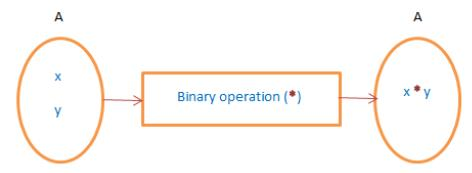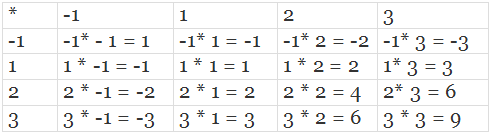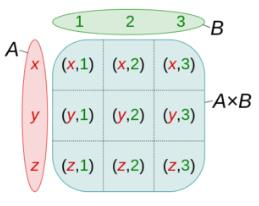# Binary Operations, Cartesian Product of Sets JEE Notes | EduRev

## Commerce : Binary Operations, Cartesian Product of Sets JEE Notes | EduRev

The document Binary Operations, Cartesian Product of Sets JEE Notes | EduRev is a part of the Commerce Course Mathematics (Maths) Class 12.
All you need of Commerce at this link: Commerce

BINARY OPERATIONS

We get a number when two numbers are either added or subtracted or multiplied or are divided. The binary operations associate any two elements of a set. The resultant of the two are in the same set. Binary operations on a set are calculations that combine two elements of the set (called operands) to produce another element of the same set.

The binary operations * on a non-empty set A are functions from A × A to A. The binary operation, *: A × A → A. It is an operation of two elements of the set whose domains and co-domain are in the same set.Addition, subtraction, multiplication, division, exponential is some of the binary operations.

Properties of Binary Operation

• Closure property: An operation * on a non-empty set A has closure property, if a ∈ A, b ∈ A ⇒ a * b ∈ A.
• Additions are the binary operations on each of the sets of Natural numbers (N), Integer (Z), Rational numbers (Q), Real Numbers(R), Complex number(C).

The additions on the set of all irrational numbers are not the binary operations.

• Multiplication is a binary operation on each of the sets of Natural numbers (N), Integer (Z), Rational numbers (Q), Real Numbers(R), Complex number(C).

Multiplication on the set of all irrational numbers is not a binary operation.

• Subtraction is a binary operation on each of the sets of Integer (Z), Rational numbers (Q), Real Numbers(R), Complex number(C).

Subtraction is not a binary operation on the set of Natural numbers (N).

• A division is not a binary operation on the set of Natural numbers (N), integer (Z), Rational numbers (Q), Real Numbers(R), Complex number(C).
• Exponential operation (x, y) → xy is a binary operation on the set of Natural numbers (N) and not on the set of Integers (Z).

Properties of Binary Operations
Commutative
A binary operation * on a set A is commutative if a * b = b * a, for all (a, b) ∈ A (non-empty set). Let addition be the operating binary operation for a = 8 and b = 9, a + b = 17 = b + a.

Associative
The associative property of binary operations hold if, for a non-empty set A, we can write (a * b) *c = a*(b * c). Suppose N be the set of natural numbers and multiplication be the binary operation. Let a = 4, b = 5 c = 6. We can write (a × b) × c = 120 = a × (b × c).

Distributive
Let * and o be two binary operations defined on a non-empty set A. The binary operations are distributive if a*(b o c) = (a * b) o (a * c) or (b o c)*a = (b * a) o (c * a). Consider * to be multiplication and o be subtraction. And a = 2, b = 5, c = 4. Then, a*(b o c) = a × (b − c) = 2 × (5 − 4) = 2. And (a * b) o (a * c) =  (a × b) − (a × c) = (2 × 5) − (2 × 4) = 10 − 6 = 2.

Identity
If A be the non-empty set and * be the binary operation on A. An element e is the identity element of a ∈ A, if a * e = a = e * a. If the binary operation is addition(+), e = 0 and for * is multiplication(×), e = 1.

Inverse
If a binary operation * on a set A which satisfies a * b = b * a = e, for all a, b ∈ A. a-1 is invertible if for a * b = b * a= e, a-1 = b. 1 is invertible when * is multiplication.
Note: 1. Identity element is unique for whole given set A(if exists) i.e each element of given set A has same identity element.
2. But inverse element is different for different elements(if exists) , but unique.

Solved Example
Q.1. Show that division is not a binary operation in N nor subtraction in N.
Solution. Let a, b ∈ N
Case 1: Binary operation * = division(÷)
–: N × N→N given by (a, b) → (a/b) ∉ N (as 5/3 ∉ N)
Case 2: Binary operation * = Subtraction(−)
–: N × N→N given by (a, b)→ a − b ∉ N (as 3 − 2 = 1 ∈ N but 2−3 = −1 ∉ N).

Q.2. Let A = {−1, 1, 2, 3}. Construct the binary table corresponding to the binary operation “multiplication” on A.
Solution. We have the following binary (or composition) table:Cartesian Product of Sets
Before getting familiar with this term, let us understand what does Cartesian mean. Remember the terms used when plotting a graph paper like axes (x-axis, y-axis), origin etc. For example, (2, 3) depicts that the value on the x-plane (axis) is 2 and that for y is 3 which is not the same as (3, 2).
The way of representation is fixed that the value of the x coordinate will come first and then that for y (ordered way). Cartesian product means the product of the elements say x and y in an ordered way.Cartesian Product of Sets
The Cartesian products of sets mean the product of two non-empty sets in an ordered way. Or, in other words, the collection of all ordered pairs obtained by the product of two non-empty sets. An ordered pair means that two elements are taken from each set.
For two non-empty sets (say A & B), the first element of the pair is from one set A and the second element is taken from the second set B. The collection of all such pairs gives us a Cartesian product.
The Cartesian product of two non-empty sets A and B is denoted by A × B. Also, known as the cross-product or the product set of A and B. The ordered pairs (a, b) is such that a ∈ A and b ∈ B. So, A × B = {(a,b): a ∈ A, b ∈ B}. For example, Consider two non-empty sets A = {a1, a2, a3} and B = {b1, b2, b3}

Cartesian product A×B = {(a1, b1), (a1, b2), (a1, b3), ( a2, b1), (a2, b2),(a2, b3), (a3, b1), (a3, b2), (a3, b3)}.
It is interesting to know that (a1, b1) will be different from (b1, a1). If either of the two sets is a null set, i.e., either A = Φ or B = Φ, then, A × B = Φ i.e., A × B will also be a null set

Number of Ordered Pairs
For two non-empty sets, A and B. If the number of elements of A is h i.e., n(A) = h & that of B is k i.e., n(B) = k, then the number of ordered pairs in Cartesian product will be n(A × B) = n(A) × n(B) = hk.

Solved Example
Q.1. Let P & Q be two sets such that n(P) = 4 and n(Q) = 2. If in the Cartesian product we have (m, 1), (n, -1), (x, 1), (y, -1). Find P and Q, where m, n, x, and y are all distinct.
Solution. P = Set of first elements = {m, n, x, y} and Q = Set of second elements = {1, -1}

Offer running on EduRev: Apply code STAYHOME200 to get INR 200 off on our premium plan EduRev Infinity!

## Mathematics (Maths) Class 12

209 videos|222 docs|124 tests

,

,

,

,

,

,

,

,

,

,

,

,

,

,

,

,

,

,

,

,

,

,

,

,

;